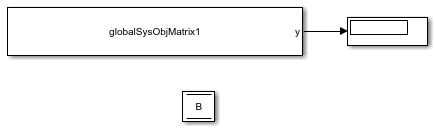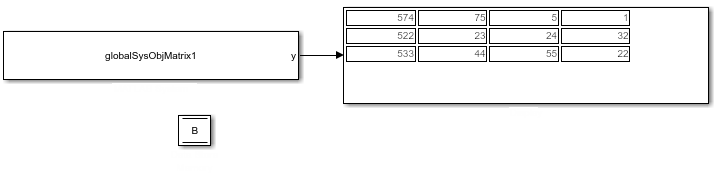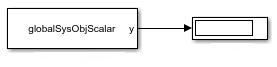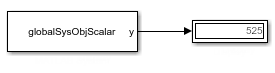## Share Data with Other Blocks

Share data between MATLAB System and other blocks using the `global` keyword and the Data Store Memory block or `Simulink.Signal` object. You might need to use global data with a MATLAB System block if:

• You have an existing model that uses a large amount of global data, you are adding a MATLAB System block to this model, and you want to avoid cluttering your model with additional inputs and outputs.

• You want to scope the visibility of data to parts of the model.

### Data Sharing with the MATLAB System Block

In Simulink®, you store global data using data store memory. You implement data store memory using either Data Store Memory blocks or `Simulink.Signal` objects. How you store global data depends on the number and scope of your global variables.

#### Scoping Rules for Shared Data in MATLAB System Blocks

The MATLAB System block uses these scoping rules:

• If you use the same name for both Data Store Memory block and `Simulink.Signal` object, Data Store Memory block scopes the data to the model.

• A global variable resolves hierarchically to the closest Data Store Memory block with the same name in the model. The same global variable appearing in two different MATLAB System blocks might resolve to different Data Store Memory blocks depending on the hierarchy of the model. You can use this ability to scope the visibility of data to a subsystem.

#### Using Shared Data in MATLAB System Blocks

MATLAB System blocks support data store memory for:

• MATLAB® structures (buses)

• Enumerated data types

#### How to Use Data Sharing with the MATLAB System Block

To use shared data in your MATLAB System block:

1. Declare a global variable in the System object™ that you associate with the MATLAB System block.

You can use the `global` keyword in these methods of the System object:

2. Add a Data Store Memory block or `Simulink.Signal` object that has the same name as the global variable in the System object.

To share data between referenced models using the `Simulink.Signal` object, define the `Simulink.Signal` object in the base workspace and use the same global variable name as in the MATLAB System block.

### Choose How to Store Shared Data

You can use Data Store Memory blocks or `Simulink.Signal` objects to store shared data.

Type of DataGlobal Data Storage MethodRelated Links

A small number of global variables in a single model that does not use model reference.

Data Store Memory blocks.

Note

Using Data Store Memory blocks scopes the data to the model.

A large number of global variables in a single model that does not use model reference.

`Simulink.Signal` objects defined in the model workspace. `Simulink.Signal` objects offer these advantages:

• You do not have to add numerous Data Store Memory blocks to your model.

• You can load the `Simulink.Signal` objects in from a MAT-file.

Data shared between multiple models (including referenced models).

`Simulink.Signal` objects defined in the base workspace

Note

If you use Data Store Memory blocks as well as `Simulink.Signal`, note that using Data Store Memory blocks scopes the data to the model.

### How to Use Data Store Memory Blocks for the MATLAB System Block

1. Declare a global keyword in the System object methods that support globals. For example:

`global A;`

3. Double-click the MATLAB System block and associate the System object.

4. Add a Data Store Memory block to your model and set:

1. Data store name to match the name of the global variable in your MATLAB System block code.

2. Data type to an explicit data type.

The data type cannot be `auto`.

3. Signal type.

4. Initial value.

The initial value of the Data Store Memory block cannot be unspecified.

#### Use Data Store Memory with the MATLAB System BlockThis model demonstrates how a MATLAB System block uses the global data stored in Data Store Memory block `B`. The MATLAB System block is associated with the `globalSysObjMatrix1` System object. To see the completed model, open the `ex_globalsys_objmatrix1` model.

1. Drag these blocks into a new model:

• MATLAB System

• Data Store Memory

• Display

2. Create a System object to associate with the MATLAB System block. To start, from the MATLAB System block, create a Basic System object template file.

3. In MATLAB Editor, create a System object with code like the following. Save the System object as `globalSysObjMatrix1.m`. The System object modifies `B` each time it executes.

```classdef globalSysObjMatrix1 < matlab.System % Global/DSM support scalar example methods(Access = protected) function setupImpl(obj) % Perform one-time calculations, such as computing constants end function y = stepImpl(obj) global B; B(:,1)= B(:,1)+100; y = B; end end end```
4. Double-click the MATLAB System block and associate the `globalSysObjMatrix1` System object with the block.

5. In the model, double-click the Data Store Memory block `B`.

6. In the Signal Attributes tab, enter an initial value, for example:

`[74 75 5 1;22 23 24 32;33 44 55 22]`
7. Simulate the model.

The MATLAB System block reads the initial value of global data stored in `B` and updates the value of `B` each time it executes. This model executes for five time steps.8. Save and close your model.

### How to Set Up `Simulink.Signal` Objects

Create a `Simulink.Signal` object in the model workspace.

Tip

Create a `Simulink.Signal` object in the base workspace to use the global data with multiple models.

1. In the Model Explorer, navigate to `model_name` > Model Workspace in the Model Hierarchy pane.

2. Ensure that these settings apply to the `Simulink.Signal` object:

1. Set Data type to an explicit data type.

The data type cannot be `auto`.

2. Set Dimensions to be fully specified.

The signal dimensions cannot be `-1` or inherited.

3. Set the Complexity.

4. Specify an Initial value.

The initial value of the signal cannot be unspecified.

5. Set Name to the name of the global variable.

#### Use a `Simulink.Signal` Object with a MATLAB System BlockThis simple model demonstrates how a MATLAB System block uses a `Simulink.Signal` with signal B. The MATLAB System block is associated with the `globalSysObjScalar` System object. To see the completed model, open the `ex_globalsys_simulink_signal_share` model.

1. Drag these blocks into a new model:

• MATLAB System

• Display

2. Create a System object to associate with the MATLAB System block. To start, from the MATLAB System block, create a Basic System object template file.

3. In MATLAB Editor, create a System object. Save the System object as `globalSysObjScalar.m`. The System object modifies `B` each time it executes.

```classdef globalSysObjScalar < matlab.System % Global/DSM support scalar example methods(Access = protected) function setupImpl(obj) % Perform one-time calculations, such as computing constants end function y = stepImpl(obj) global B; B= B+100; y = B; end end end```
4. Double-click the MATLAB System block and associate the `globalSysObjScalar` System object with the block.

5. From the model, on the Modeling tab, click Model Explorer.

6. In the left pane of the Model Explorer, select the model workspace for this model.

The Contents pane displays the data in the model workspace.

7. In the Model Explorer, in the Model Hierarchy pane, navigate to `model_name` > Model Workspace. In the Contents pane, set Name to `B`.

8. Navigate back to `model_name` > Model Workspace.

• Make these settings for the `Simulink.Signal` object:

AttributeValue
Data type`double`
Complexity`real`
Dimensions`1`
Initial value`25`
9. Simulate the model.

The MATLAB System block reads the initial value of global data stored in `B` and updates the value of `B` each time it executes. The model runs for five time steps.10. Save and close your model.

### Using Data Store Diagnostics to Detect Memory Access Issues

You can configure your model to provide run-time and compile-time diagnostics to avoid problems with data stores. Diagnostics are available in the Configuration Parameters dialog box and the Parameters dialog box for the Data Store Memory block. These diagnostics are available for Data Store Memory blocks only, not for `Simulink.Signal` objects. For more information on using data store diagnostics, see Data Store Diagnostics.

### Limitations of Using Shared Data in MATLAB System Blocks

The MATLAB System block does not support data store memory for variable-sized data

### Use Shared Data with P-Coded System Objects

If the System object is P-code, you must implement the `getGlobalNamesImpl` method to provide the global variable names you use in the System object. For example:

```classdef GlobalSysObjMatrix < matlab.System % Matrix DSM support: Increment first row by 1 at each time step methods (Access = protected) function y = stepImpl(obj) global B; B(1,:) = B(1,:)+1; y = B; end function globalNames = getGlobalNamesImpl(~) globalNames = {'B'}; end end end```